Courses
Courses for Kids
Free study material
Offline Centres
More

# Circumcircle of a TriangleLast updated date: 01st Dec 2023
Total views: 125.7k
Views today: 1.25k## Let’s Know More About Circumcircle

A plane figure in which a point is taken as the centre and from that point dots which are equidistant are marked and then joined which makes a circle. The first point or the reference point is known as a centre and the distance between the centre and the surrounding path is known as the radius of the circle.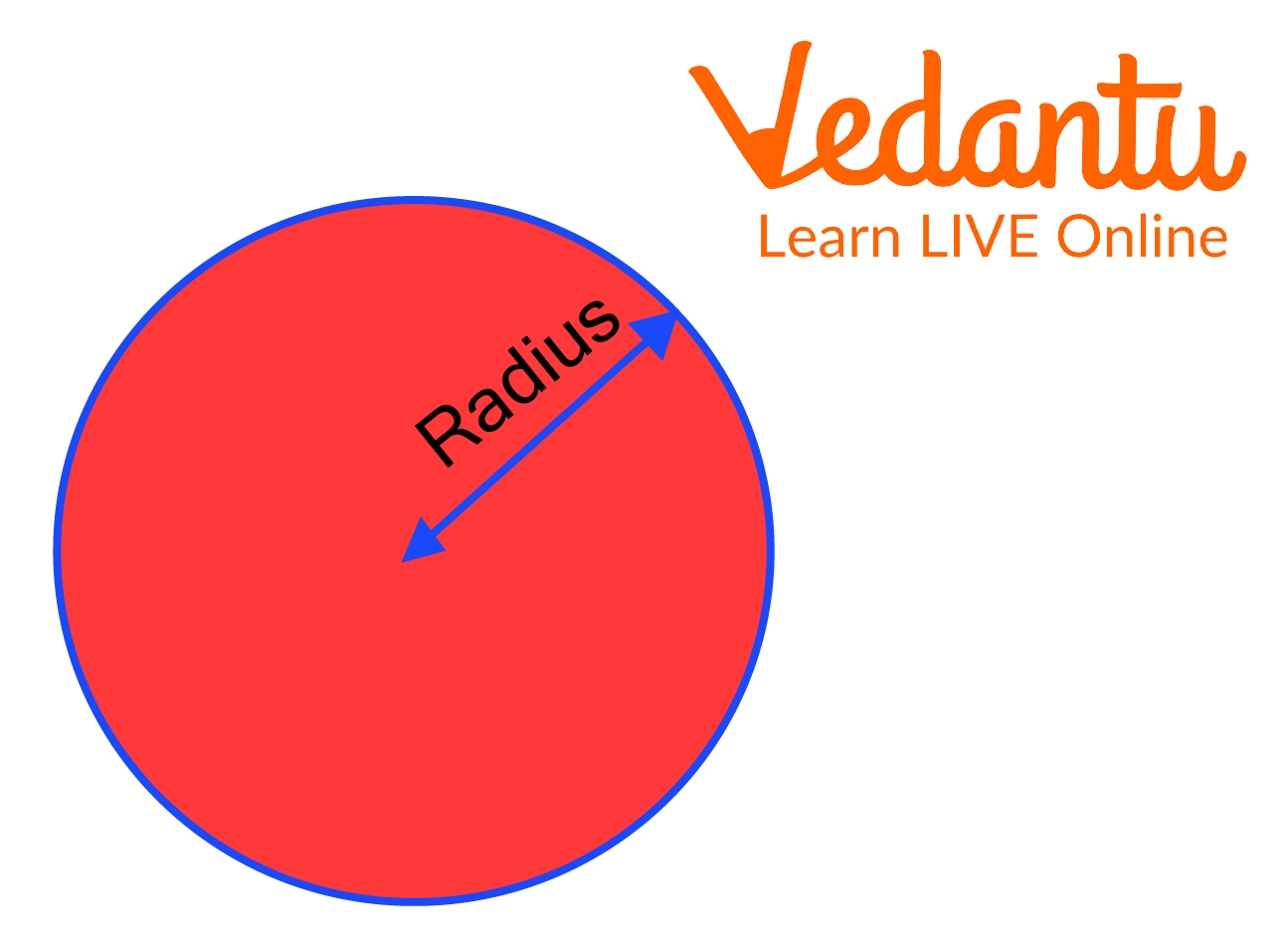The circle which encircles a polygon by touching each vertex of the polygon is termed a circumcircle. It is drawn by taking the meeting point of the perpendicular bisector to the sides of the polygon as the centre.

## What is Circumcircle?

A circumcircle is a circle which circumscribes any polygon and touches each vertex of the polygon. The radius of the circumcircle is the distance between the point of meeting of bisectors and the vertex. The circumcircle is drawn by taking the meeting point of the perpendicular bisector to the sides of the polygon as the centre.

## The Circumcircle of a Triangle Formula

The circumcircle of a triangle can be simply derived using the following various methods. The formula is not universal since it changes based on the type of triangle. So let’s find out more about how to find the circumcircle of a triangle.

## The Circumcircle of a Triangle

For example, we have to draw a circumcircle to the given triangle then the following steps must be followed;

Step 1: Draw the perpendicular bisectors of the sides.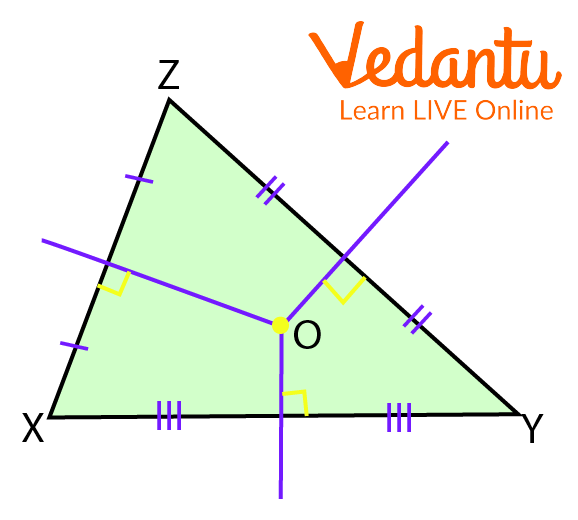Circumcircle around a Triangle

Step 2: Taking point O as the centre we draw a circle which touches each vertex of the triangle.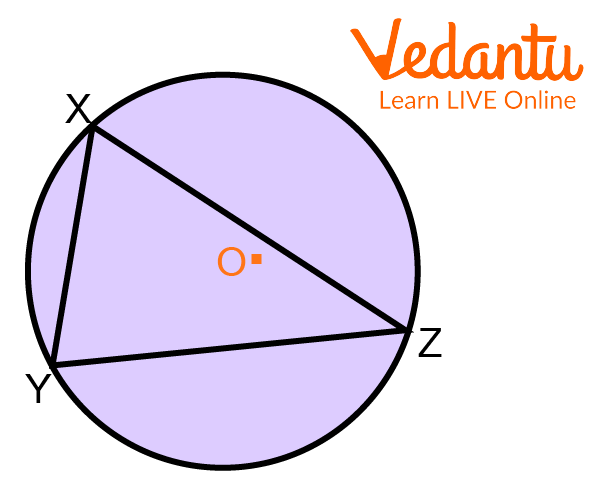Process of Drawing a Circumcircle Around a Triangle

Step 3: The distance OZ, OX and OY is said to be the circumradius.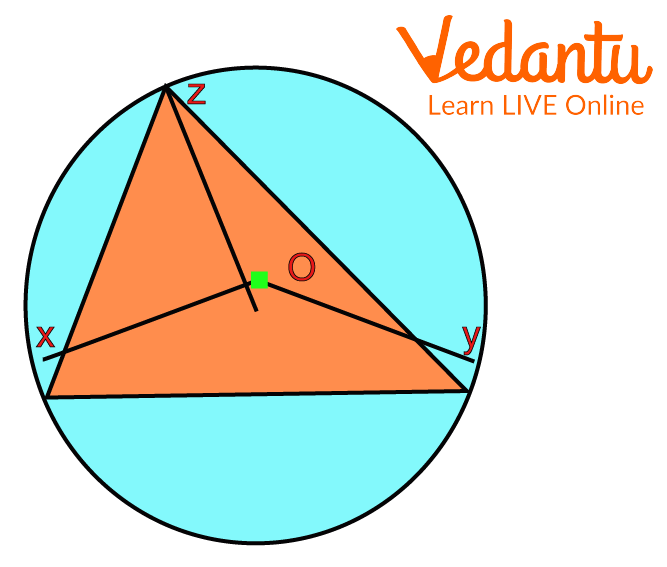Circumcentre

Hence, the required circumcircle is as above with the circumcentre as O.

## Circumradius of Right Angle Triangle

The Circumradius of the right angle triangle is the radius of the circle of the circumcircle which is drawn around a polygon. Let us draw a circumcircle for the right-angled triangle.

Step 1: Take a right-angled triangle.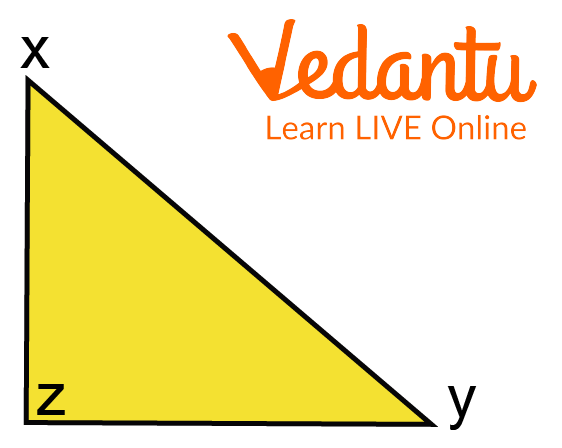Right-angled Triangle

Step 2: Draw the perpendicular bisector of the sides of the triangle.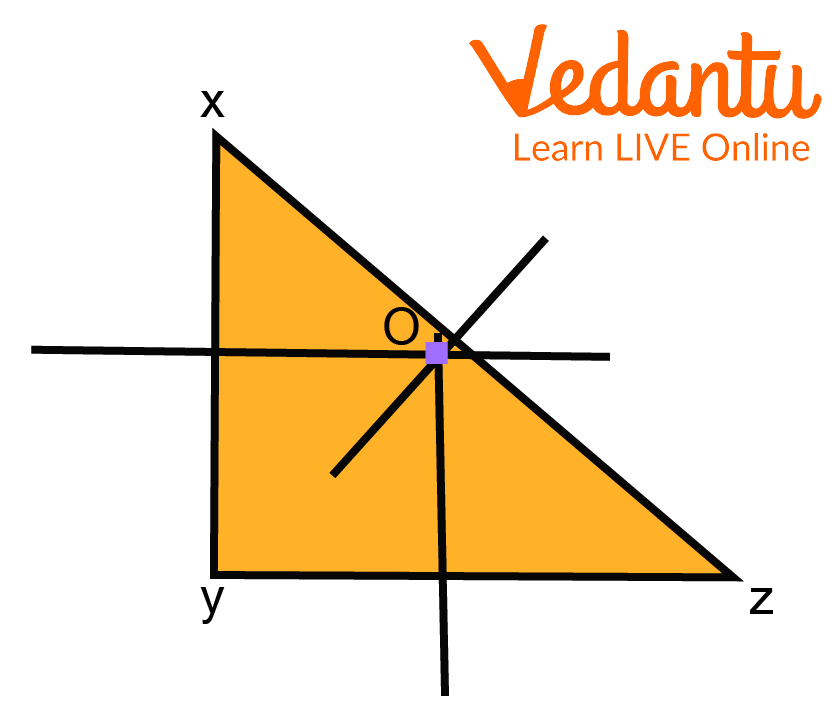Perpendicular Bisector

Step 3: Taking the meeting point of the perpendicular bisector and the vertex which is most distant from it as a radius, draw a circle which passes through each vertex of the triangle.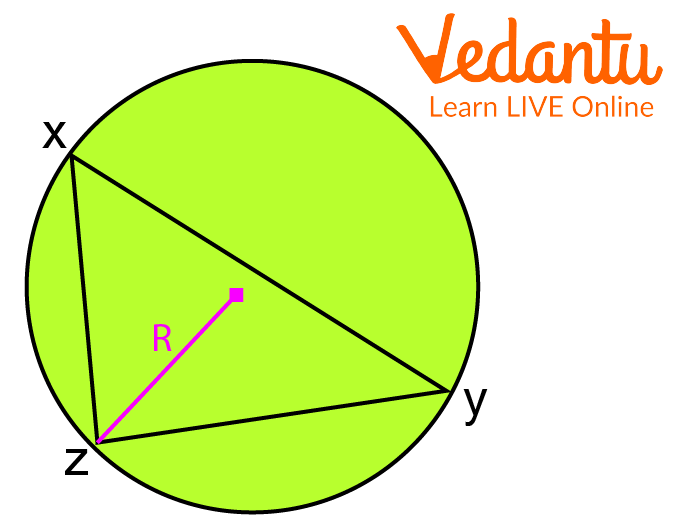Right-angled Triangle in a Circumcircle

Hence, the circumcircle of the given right-angled triangle with circumradius “R” is as depicted in the figure above.

## How to find the Circumradius of a Triangle?

Let that there is a circumcircle which is circumscribing a triangle with sides a, b and c with a circumcentre.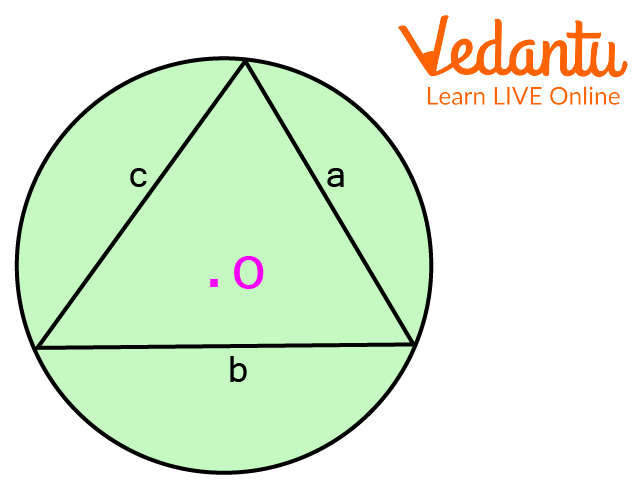Circumcircle Circumscribing a Triangle

$2 \mathrm{R}=\dfrac{a}{\operatorname{Sin} A}=\dfrac{b}{\operatorname{Sin} B}=\dfrac{c}{\sin C}$

Now, Area of triangle $\left(A_{t}\right)=\dfrac{1}{2} a b \operatorname{Sin} C$

$\operatorname{Sin} C=\dfrac{C}{2 R}$

Therefore, $\mathrm{A}_{\mathrm{t}}=\dfrac{1}{2} a b \dfrac{C}{2 R}$.

Now, $\mathrm{R}=\dfrac{a b c}{4 A}$

Hence, the required formula to find the circumradius of the triangle is $\mathrm{R}=\dfrac{a b c}{4 A}$.

## Solved Questions

Q1. List all the steps in drawing a circumcircle of a triangle.

Ans: To draw a circumcircle of a triangle follows the steps below:

Step 1: Draw the perpendicular bisectors of the sides

Step 2: Taking point O as the centre we draw a circle which touches each vertex of the triangle.

Step 3: The distance between OZ, OX and OY is said to be the circumradius.

Q2. Draw the circumcircle of a triangle with sides of 6 cm, 8 cm and 10 cm. What is the radius of this circle?

Ans: 5 cm

Steps for construction:

Step 1: Construct ΔABC with AB = 6 cm, BC = 8 cm and CA = 10 cm.

Step 2: Draw the perpendicular bisectors of any two sides of the triangle (say, AB and BC).

Step 3: Find the point of intersection of the perpendicular bisectors and mark it as O.

Step 4: Measure the distance OA or OB or OC, they are all equal to the radius of the circumcircle.

Step 5: OA = OB = OC = 5 cm

## Practice Questions

Q1. Considering the given statement states whether it is true or false with reason: “A circle drawn inside a triangle touching all its sides is known as a circumcircle.”

Ans: False

Q2. Circumradius is the distance between the meeting point of the bisector of sides of a polygon and the vertex. True or False explain with reason.

Ans: True

Q3. Circumcentre of a circle is the point of concurrency of the

(a) angular bisectors of vertices

(b) medians

(c) perpendicular bisectors of sides

(d) any two sides

Ans: (c) perpendicular bisectors of sides

Q4. The ratio of the area of the circumcircle and incircle of an equilateral triangle is

(a) 4:1

(b) 1:4

(c) 9:1

(d) 1:9

## Summary

After reading this article you must have been very well aware of the concepts of the circumcircle, circumradius, how to draw a circumcircle of a triangle and about circles. These concepts will help in solving problems of geometry and Circles in your textbook. You have also learnt the relationship between the radius of the circumcircle and the area of the triangle. We have learnt that a circle that touches the vertex of the triangle and is drawn outside the triangle is known as a circumcircle. But when a circle touches the sides of the triangle and is drawn inside the triangle it is known as a circle. The distance between the meeting point of the bisector of sides and the vertex is known as the circumradius.

## FAQs on Circumcircle of a Triangle

1. What do you understand by the term circumradius?

The radius of the circle circumscribing a polygon is known as the circumradius of the circle. It is the distance between the meeting point of the perpendicular bisector of the sides and the vertex of the polygon.

2. What do you understand about the term right-angled triangle?

A triangle in which one of the angles is 90⁰ is known as a right-angled triangle. In a right-angle triangle, the sum of the squares of two smaller sides is always equal to the square of the largest side. This theorem was given by Pythagoras and hence named Pythagoras theorem.

3. What is a Circumcenter? Does Every Triangle have a Circumcenter?

The circumcenter is the intersection point of the perpendicular bisectors of the sides of a triangle. It is the centre of a triangle’s circumcircle. Every triangle has a circumcenter. The circumcenter can be either inside the triangle or outside.